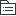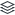# 公共基础知识-公文写作题

|
32

## 相关模板推荐

•## 公文写作 —— 作品大纲

•公文概述
• 特点
• 公务性
• ☆规范性
• 规范体式
• 格式：依据官方文件
• ☆语言表达：准确、简洁、庄重、严谨
• ☆文体：说明、叙述、议论
• 法定性
• 作者法定
• 发文机关（撰写人）
• 程序性
• 权威性
• 强制性
• 公文区别于其他文书的最大特点
• ☆时效性
• 及时有期限、时代性
• 文稿
• 正本
• 有效
• 副本
• 仅复印
• 无效
• 复印+盖章
• 有效
• 试行本
• 有效（一定范围后全部范围，将来长期性）
• 暂行本
• 有效（一定时间内，暂时性，一般为紧急）
• 修订本
• 有效
•公文格式
• ☆版头
• 首页，红色分割线以上
• 份号
• 6位数字，涉密公文才标
• 密级和保密期限
• 保密期限
• 阿拉伯数字表示
• 密级
• 绝密
• 最重要
• 30年
• 机密
• 重要
• 20年
• 秘密
• 一般性
• 10年
• 紧急程度
• 程度
• 公文
• 特急、加急
• 电报
• 特提、特急、加急、平急
• ☆发文机关标志
• 发文机关全程/规范化简称+文件（可省）
• 联合发布
• 主办在前、协办在后
• 只写主办方
• ☆发文字号
• 发文机关代字
• 国办发√
• 没有国办字的说法
• 关系
• 上级发下级，xx发
• 下级发上级，xx呈
• 同级之间，xx函
• 年份
• 4位，用六角括号
• 发文顺序
• 不加“第”，不编虚位（不加0），xx号
• 签发人
• 只有上行文时写
• 签发人：xxx xxx（一排2）
• 发文字号左空一格，且与最后一个签发人同一行
• 主体
• 标题
• 构成
• 发文机关名称
• 事由
• 文种
• 不可省略
• 回行
• 词意完整，排列对称，长短适宜，间距恰当，菱形或梯形
• 标题
• 不可出现
• 逗号
• 只有一个
• 关于
• 引用其他公文或法律法规
• 书名号
• 出现特定含义的词语
• 引号
• 主送机关
• 全称、规范化简称、同类机关统称
• 先高后低
• 各市、县人民政府
• 先外后内
• 党政军群
• 第一页要出现正文
• 主送机关过多时移至版记
• ☆正文
• 序号
• 第一层
• 一、
• 第二层
• （一）
• 第三层
• 1.
• 第四层
• （1）
• 语句
• 常见病句：成分赘余、用语不准确、搭配不当、语句歧义、色彩不当
• 要求：准确简洁无歧义，色彩搭配要恰当
• 引用
• ☆先引用标题、后引用发文字号，发文字号外面加括号
• 结语
• 特此函询
• 如蒙惠允，不胜感谢
• 请示
• 当否，请批示
• 妥否，请批示
• 公文附件的顺序号和名称
• 只有一个附件，不标序号
• 附件名称后不加标点符号（《》，。都不加）
• 附件名称过长和回行时与上一行首字对其
• 落款
• 发文机关署名
• 全称、简称都可以
• ☆成文日期
• 格式
• 右空4字
• 阿拉伯数字+年月日，数字不编虚位
• 日期
• 会议
• 会议通过当天发
• 当天
• 会议讨论，非当天发
• 发布当天
• 负责人签发
• 联合行文，最后签发日期
• 印章
• 上不压正文，下压日期
• 联合行文，都要署名盖章
• 附注
• 文件发放、传达方式
• 抄送机关
• 同一系统同级机关
• 顿号
• 不同系统机关
• 逗号
• 最后一个
• 句号
• 附件
• 版记
• 版记中无“主题词”要素
• 抄送机关指需要了解、知晓公文内容的机关
• 印发机关一般只发文机关的办公厅（室）（省政府办公厅、市政府办公室）
• 印发在成文之后
• 页码
• 单页码
• 居右，空一字
• 双页码
• 居左，空一字
• 字体字号
• 一般
• 3号仿宋
• 密级、紧急程度
• 3号黑体
• 发文机关标志
• 小标宋体
• 标题
• 2号小标宋体
• 签发人姓名
• 3号楷体，“签发人3字”：3号仿宋
• 抄送、印发机关、日期
• 4号仿宋
•公文的种类和分类
• 种类
• 决议
• 会议讨论
• 决定
• 对重要事项作出决定和部署
• 变更或撤销下级机关不适当的决定事项
• ☆奖惩有关单位或人员
• 不属于同一系统，长期做出贡献
• 命令
• 公布行政法规
• 嘉奖有关单位
• 只嘉奖不惩罚
• 嘉奖在突发事件中做出贡献的人
• 公报
• 特点：新闻性、报道性
• 公告
• 国内外
• 宣布重要实行或法定事项
• 通告
• 一定范围内
• 发布应当遵守或者周知的事项
• 意见
• 对重要事项提出见解和处理办法
• 多向性，上下平
• 通知
• 称号
• 公文之王、公文中的轻骑兵
• 适用
• 发布、传达，上级要求下级执行
• 批转
• 转发
• 通报
• 表彰先进、批评错误
• 表彰同系统内的下级机关
• 传达重要的精神和告知重要情况
• 报告
• 上行文
• 事前、事中、事后都可以发
• 请示
• 上行文
• 只能事前请示
• 期待上级的批复
• 批复
• 下行文
• 答复下级机关的请示事项
• 议案
• 各级人民政府向同级人大、人常提出
• 不相隶属的机关之间
• 纪要
• 记载会议主要情况
• 没有“会议纪要”的说法
• 记载全部
• 记录
• 分类
• 行文方向
• 上行文
• 报告、请示
• 下行文
• 命令、决定
• 平行文
• 函、议案
• 内容
• 法规性、指挥性、知照性、报请性、记录性
•公文的行文规则
• 基本
• 不得越级行文
• 可以越级
• 事情特别重大紧急
• 多请未决
• 上上级指定越级
• 向上级，上行文
• 主送一个上级机关，不抄送下级
• 提出倾向性意见后上报
• 请示应当一文一事
• 机关对机关
• 双重领导，必要时，抄送另一个上级机关
• 向下级，下行文
• 重要行为抄送给发文机关的直接上级
• 办公厅室经授权，可以发指令性要求
• 涉及多部门，协商一致
• 双重领导，必要时抄送另一个上级机关
• 特殊
• 同级可以联合行文（各自职权范围内的工作不得联合行文）
• 党委政府的部门可以依据职权相互行文
•公文的拟制和办理
• 拟制
• 原则
• 流程
• 起草
• 审核
• 签发
• 普通
• 负责人（副职）
• 重要
• 主要负责人（正职）
• 联合行文
• 会签
• 圈阅
• 签名
• 办理
• 发文办理
• 复核-登记-印制-核发
• 收文办理
• 签收-登记-初审-承办-传阅-催办-答复拟办（初步拟定）、批办（领导批示）、注办（做标注）
• 归档
• 有价值
• 原件-主办机关
• 复制件-相关机关
• 无价值
• 销毁
• 重大错漏
• 清退
• 管理
• 绝密公文
• 一般不得复制、汇编
• 复制汇编的，视同原件管理
• 复制件
• 加盖复制机关戳记（盖章）
• 汇编本的密级
• 按照最高密级标注
• 新设立机关
• 向本级党委、政府的办公厅室提出发文立户申请